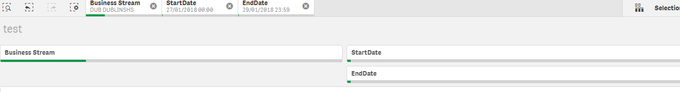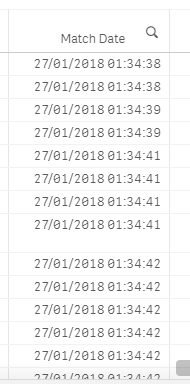# New to Qlik Sense

If you’re new to Qlik Sense, start with this Discussion Board and get up-to-speed quickly.

Announcements
cancel
Showing results for
Did you mean:Contributor III

## selection based on two timestamps

Hi all,

I Created Startdate and EndDate variables using the below definitions.

vStartDate1 as "=max({<StartDate = {"\$(=min(StartDate))"}>} StartDate)"

vEndDate1 as "=Min({<EndDate = {"\$(=min(EndDate))"}>} EndDate)"

I have selected dates as below but the data is not getting filtered correctly.here is the expression used for match date in the table.

=if(timestamp(timestamp#([Match Date],'DD/MM/YYYY hh:mm:ss'),'DD/MM/YYYY hh:mm')>='\$(vStartDate1)' and timestamp(timestamp#([Match Date],'DD/MM/YYYY hh:mm:ss'),'DD/MM/YYYY hh:mm')<='\$(vEndDate1)',[Match Date])

here is the script that I used to generate data from 2017.

let vEndDate=Timestamp(now(),'YYYY-MM-DD hh:mm:ss');
trace \$(vmindate);

LET vMinDate = num(Timestamp(Timestamp#(now()))-1468);

LET vMaxDate = num(Timestamp(Timestamp#(now())));

LET vNumberOfDays = Floor(\$(vMaxDate)) - Floor(\$(vMinDate)) + 1;

Calendar_Date_1Months:

Timestamp(Num(\$(vMaxDate)) - (RecNo())/24/60 ) as Date,
Num(Floor(Date(Num(\$(vMaxDate)) - RecNo()/24/60 ))) as DateKey
AutoGenerate 24*60* \$(vNumberOfDays) ;

StartDate:

Timestamp((Date),'DD/MM/YYYY hh:mm') as StartDate
Resident Calendar_Date_1Months
order by DateKey desc;

EndDate:

Timestamp((Date),'DD/MM/YYYY hh:mm') as EndDate
Resident Calendar_Date_1Months
order by DateKey desc;

MatchDate is in timestamped format.Thanks

Sujana

Labels (1)
• ### General Question

1 Solution

Accepted SolutionsMVP

If you are already selecting a single Start Date and a single EndDate, why do you need set analysis in your variable?... I say try this

``````vStartDate1 as "=max(StartDate)"
vEndDate1 as "=Min(EndDate)"``````

And then may be this

``=If([Match Date] >= \$(vStartDate1) and [Match Date] <= \$(vEndDate1), [Match Date])``

or just this

``=If([Match Date] >= [Start Date] and [Match Date] <= [End Date], [Match Date])``
2 RepliesMVP

If you are already selecting a single Start Date and a single EndDate, why do you need set analysis in your variable?... I say try this

``````vStartDate1 as "=max(StartDate)"
vEndDate1 as "=Min(EndDate)"``````

And then may be this

``=If([Match Date] >= \$(vStartDate1) and [Match Date] <= \$(vEndDate1), [Match Date])``

or just this

``=If([Match Date] >= [Start Date] and [Match Date] <= [End Date], [Match Date])``Contributor III
Author

Thanks so much!! did the same.